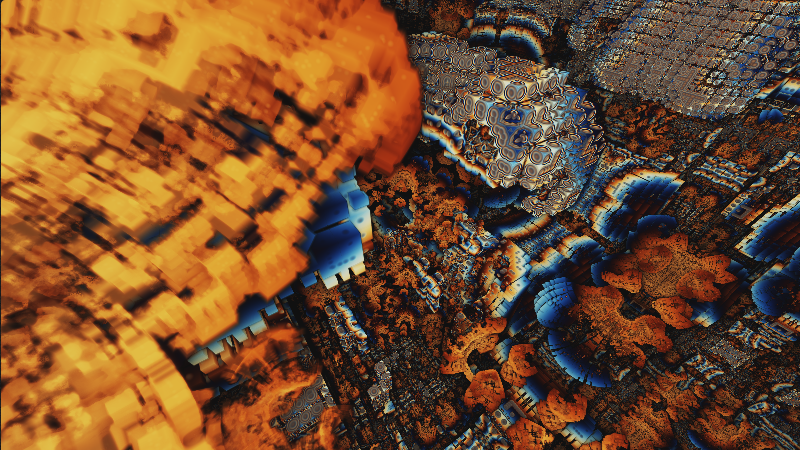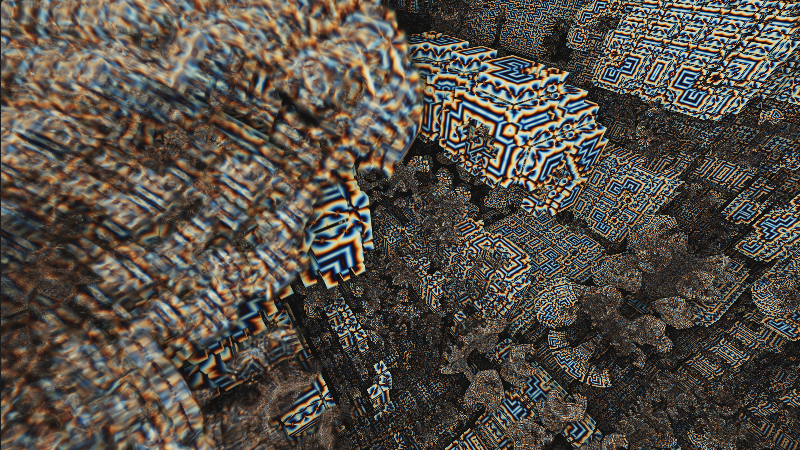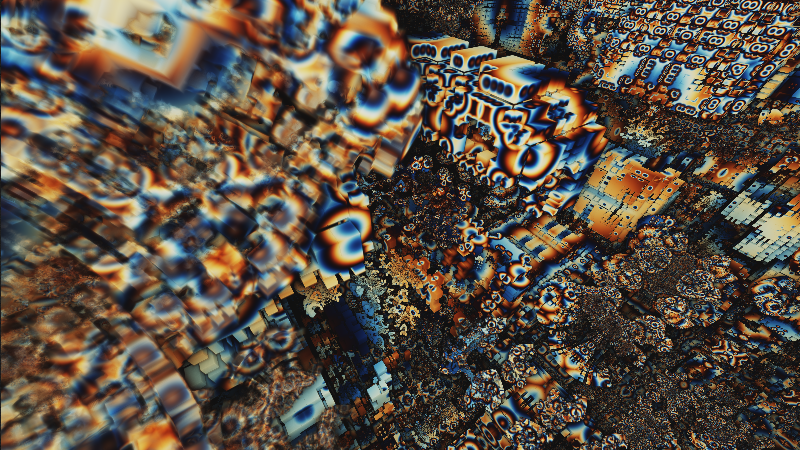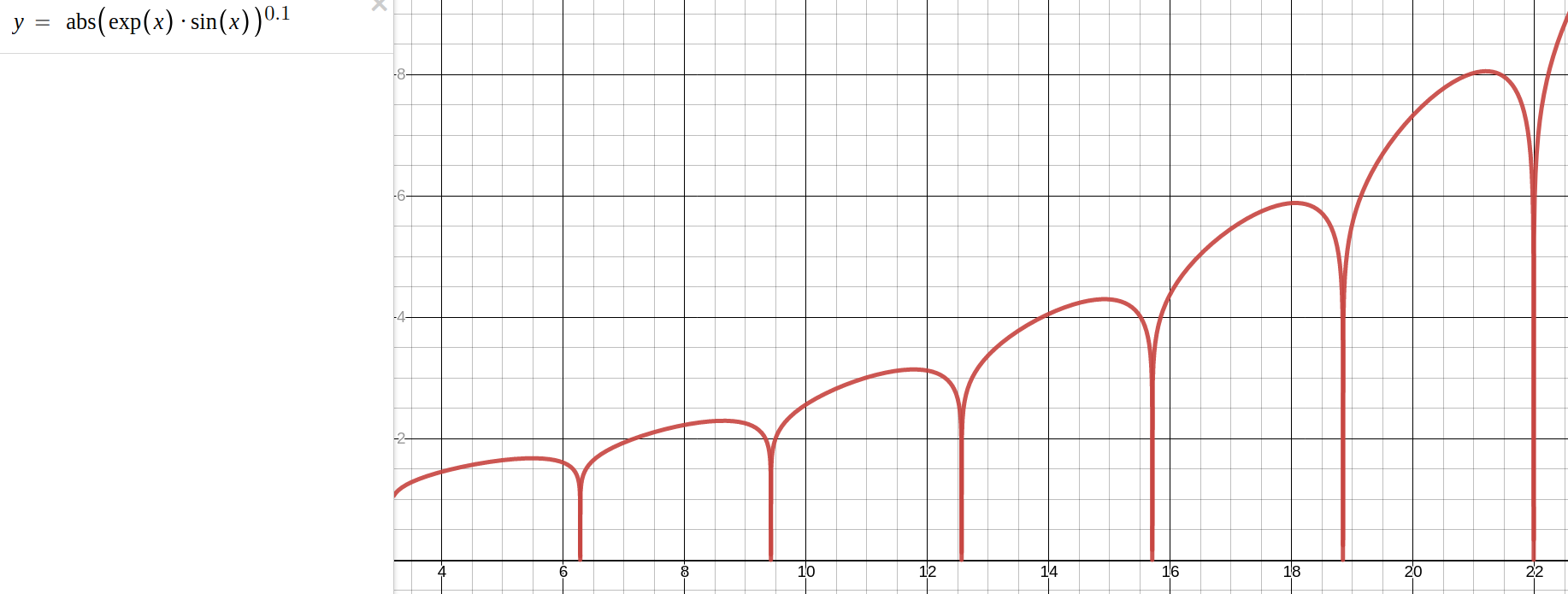# Jon Baker, Graphics Programming

## Orbit Traps

You can use the orbit trap method to color these fractal distance estimators. When you are evaluating a transformed point over and over iteratively in a loop, as is the case for some of these fractal distance estimator functions, the point in question will move around the space in different ways. It is possible to use an escape counter variable, and upon evaluation of the final loop in the distance estimator, use this information to index into a palette that wraps around, in order to produce a color - I will explain a couple methods below.

There are a few qualities which you might consider when updating this palette indexing variable. I have seen two metrics used, and came up with another one, which I'm sure has been used before. The form of thes fractal distance estimators often follows a pattern of repeatedly transforming the input point, p. There is a length basis, which considers the `length(p.xyz)`, a radial basis, which considers the angle about the origin, and a metric that uses the distance from the location of p during the previous iteration of the loop.

## Length BasisLength basis is very simple - a single function call. Length of the vector, or the length squared using the dot product.

```// from Nameless
orbit_term = length(p.xyz);
-or-
orbit_term = dot(p,p);

```Radial basis requires the consideration of a few trigonometric functions, as shown here:

```  vec3 norm = normalize(p.xyz);
float theta = acos(norm.z/length(norm.xyz));
float phi = atan(norm.y/norm.x);

orbit_term = max(theta,phi); // or some other operation based on these two euler angles
```

## Displacement BasisDistance from previous point location - this requires maintainence of state, updating some `pprev` at the beinning of each loop iteration with the previous value of p, before any transforms are applied.

```  orbit_term = distance(p, pprev);
```

And once you have computed a result for this orbit term, there are a few ways to update the state. You might perform some transform on this variable, say `transformed_term = exp(-0.2*orbit_term);`, or get more creative with the formula there, maybe even something like the formula below.Once you have this transformed orbit term, you can use it in a few different ways. You may want to initially set the escape term to some large value, and each update consider `escape = min(escape, transformed_term);`. You might use a small or zero initial value for the escape term, and then consider `escape = max(escape, transformed_term);`. The most trivial method would probably be to just accumulate these values with `escape += transformed_term;` performed in each of these loop iterations.

In a raymarching context, or any collision based method, it becomes important to control this variable's state during its lifetime. My raymarcher, for example, uses the distance estimator function potentially many times on the way to determining the first hit, far more times while traversing to the various light locations, and again when evaluating the positions for the ambient occlusion term. As more and more of these types of evaluations are executed, the more and more skewed the resulting value of `escape` will become, with what will become strange artifacts resembling shadows, edge glows, and other strange behavior. It becomes important to reset the value for the escape index term at the beginning of each evaluation. The easiest way is to reset it to some minimum or maximum value at the beginning of each run of the distance estimator function, so that the escape variable only contains the result from one evaluation of the distance estimator function. Evaluated at the hit location, this is a good representation of what the color should be there.

When you have a final result for your orbit trap term, you can use something like Inigo Quilez's palette functions, which will take your final float `escape` parameter and map it to an output color. There are many other uses, such as using it to control material parameters, such as emissive or metallic properties.

Last updated 6/1/2021